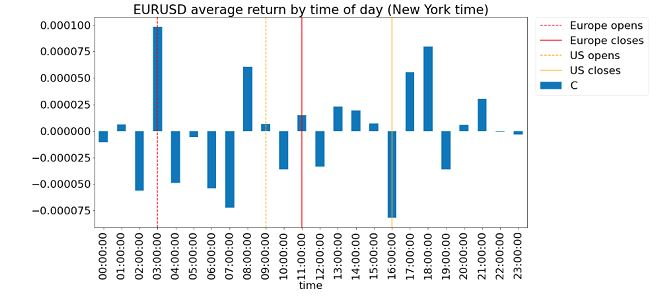## Jumat, 15 November 2013

### Cointegration Trading with Log Prices vs. Prices

In my recent book, I highlighted a difference between cointegration (pair) trading of price spreads and log price spreads. Suppose the price spread hA*yA-hB*yB of two stocks A and B is stationary. We should just keep the number of shares of stocks A and B fixed, in the ratio hA:hB, and short this spread when it is much higher than average, and long this spread when it is much lower. On the other hand, for a stationary log price spread hA*log(yA)-hB*log(yB), we need to keep the market values of stocks A and B fixed, in the ratio hA:hB, which means that at the end of every bar, we need to rebalance the shares of A and B due to price changes.

This much is easy to understand. But thanks to a reader Ferenc F. who referred me to a paper by Fernholz and Maguire, I realize there is a simple mathematical relationship between stock A and B in order for their log prices to cointegrate.

Let us start with a formula derived by these authors for the change in log market value P of a portfolio of 2 stocks: d(logP) = hA*d(log(yA))+hB*d(log(yB))+gamma*dt.

The gamma in this equation is

gamma=1/2*(hA*varA + hB*varB), where varA is the variance of stock A minus the variance of the portfolio market value, and ditto for varB.

Note that this formula holds for a portfolio of any two stocks, not just when they are cointegrating. But if they are in fact cointegrating, and if hA and hB are the weights which create the stationary portfolio P, we know that d(logP) cannot have a non-zero long term drift term represented by gamma*dt. So gamma must be zero. Now in order for gamma to be zero, the covariance of the two stocks must be positive (no surprise here) and equal to the average of the variances of the two stocks. I invite the reader to verify this conclusion by expressing the variance of the portfolio market value in terms of the variances of the individual stocks and their covariance, and also to extend it to a portfolio with N stocks. This cointegration test for log prices is certainly simpler than the usual CADF or Johansen tests! (The price to pay for this simplicity? We must assume normal distributions of returns.)

===

My online Quantitative Momentum Strategies workshop will be offered on December 2-4. Please visit epchan.com/my-workshops for registration details.